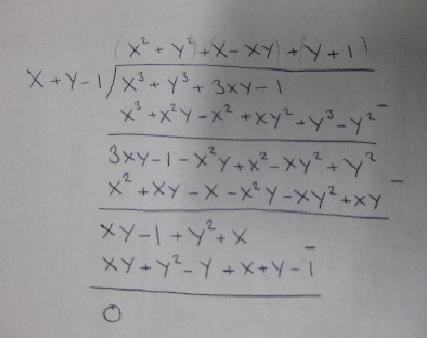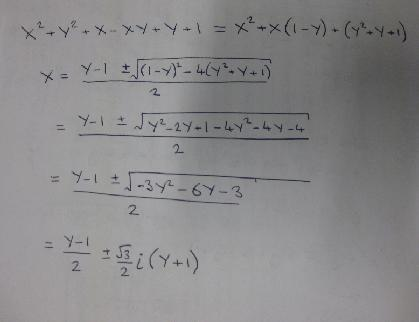#### You may also like### Power Up

Show without recourse to any calculating aid that 7^{1/2} + 7^{1/3} + 7^{1/4} < 7 and 4^{1/2} + 4^{1/3} + 4^{1/4} > 4 . Sketch the graph of f(x) = x^{1/2} + x^{1/3} + x^{1/4} -x### Parabella

This is a beautiful result involving a parabola and parallels.### Snookered

In a snooker game the brown ball was on the lip of the pocket but it could not be hit directly as the black ball was in the way. How could it be potted by playing the white ball off a cushion?

# Spot the Difference

##### Age 16 to 18 Short Challenge Level:

Chieh Lung from Kolej Tuanku Jaafar school made a good start on this:

$x + y -1 =0$ and $x + y = 1$ are the same equation, they give the top line, running through $(1,0),(0.5,0.5)$ and $(0,1)$.
$x + y = 0$ is the lower line, running through the origin.
The rest of the equations must just be multiples of these.

$(x+y-1)(x^2+y^2)=0$. The solutions of this are like solving a quadratic equation, when solutions are when at least one of the brackets is equal to zero. Here the first bracket is the top line, and the second only has solutions at $(0,0)$ as we are in the real numbers.

$(x + y)(y^2+(x+1)^2)=0$. The first bracket is the top line through the origin, and the second only has solutions at $(-1,0)$, as we are in real numbers.

$x^3 + 3xy + y^3 = 1$. This must also be a product of the lines and points. But I cannot find them.

I looked for solutions to $x^3 + 3xy + y^3 = 1$. I found $(1,0), (0.5,0.5)$ and $(0,1)$. So I thought a factor would be $x + y -1$, so I solved this with long division.So you can see that $x^3 + y^3 +3xy -1 = (x +y)(x^2+y^2+x+y-xy+1)$
To see if $x^2+y^2+x+y-xy+1$ has any solutions I wrote it as a quadratic in $x$ and used the quadratic formula.As you can see the solutions are imaginary, they contain an $i$. So The second bit can only equal zero at specifc points, not a whole line. So another point at $(-1,-1)$.
This effect could be created many times by choosing two lines, and then multiplying them by points (say $x^2+y^2=0$).